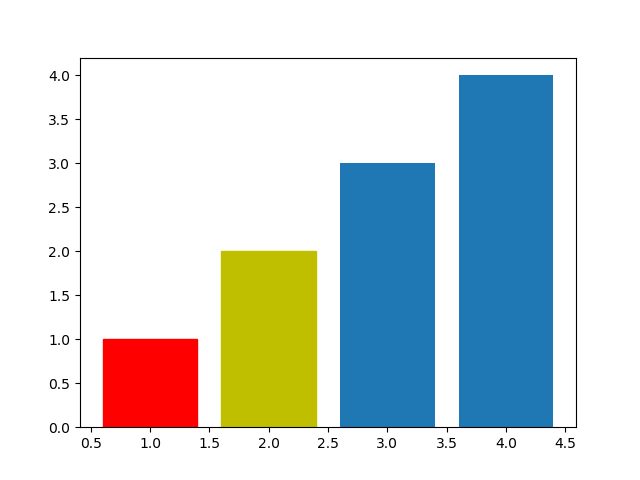## How to display a bar chart in a different color in python

When we use the bar chart in matplotlib python library, the default bar color is uniform. We can change it by simply using the set_color() property. For example, follow this code snippet- import matplotlib.pyplot as plt plt.figure() barlist=plt.bar([1,2,3,4],...## Draw/plot a line graph in python using matplotlib

Data visualization and interpretation are very important to understand the data and its property. Making decisions from raw data is really difficult especially in machine learning, deep learning, accuracy comparison, etc. Using python it is very easy to plot a graph,...